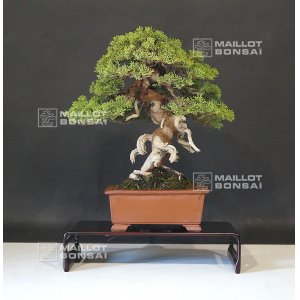##### The Japanese Bonsai specialist
Direct order Contact Help / Services Newsletter# Juniperus chinensis itoigawa ref:14080193

Juniperus chinensis itoigawaref. : 10213

1.260,00

Available quantity : 1Order

###### Description

Height of the tree without its pot: 350 mm.

Width of the branches: 370 mm.

Non-enamelled pot: 220x220x85 mm.

Moyogi style. Diameter of the trunk: 40/50 mm. Nebari: 130 mm.

This bonsaï was made from a natural sample (yamadori) and is planted in a square form pot.

Repot it in a less deep oval or round pot would highlight it even more.

Requires generous watering and a regular fertilization all along the dormancy period.

Well-ramified subject with graceful trays, a shari on the trunk and jins.

This tree does not have any strong cutting wound or wire mark.

For bonsaï's lover.

Itoigawa variety with green canopy thin scales, particularly vigorous, and perfectly adapted to the bonsaï cultivation. Unlike the classic juniperus chinensis, it only loses little foliage in the beginning of summer.

Shelf non-included.

It comes from Master Suzuki's nursery: Jusho-en nursery.

Shaped, softly ligatured and photographed in July 2019.

#bonsai 5.6 #juniperus 3.9 #chinensis 3.9 #itoigawa 3.8 #this 3.4 #coniferous 3 #nursery 2.7 #trunk 2.5 #from 2.4 #have 2.4

Formule
(( ROUND((CHAR_LENGTH(b.article_nom)-CHAR_LENGTH(REPLACE(b.article_nom, 'juniperus', '')))/LENGTH('juniperus')) + ROUND((CHAR_LENGTH(b.article_description)-CHAR_LENGTH(REPLACE(b.article_description, 'juniperus', '')))/LENGTH('juniperus')) ) * 3.9) + (( ROUND((CHAR_LENGTH(b.article_nom)-CHAR_LENGTH(REPLACE(b.article_nom, 'chinensis', '')))/LENGTH('chinensis')) + ROUND((CHAR_LENGTH(b.article_description)-CHAR_LENGTH(REPLACE(b.article_description, 'chinensis', '')))/LENGTH('chinensis')) ) * 3.9) + (( ROUND((CHAR_LENGTH(b.article_nom)-CHAR_LENGTH(REPLACE(b.article_nom, 'itoigawa', '')))/LENGTH('itoigawa')) + ROUND((CHAR_LENGTH(b.article_description)-CHAR_LENGTH(REPLACE(b.article_description, 'itoigawa', '')))/LENGTH('itoigawa')) ) * 3.8) + (( ROUND((CHAR_LENGTH(b.article_nom)-CHAR_LENGTH(REPLACE(b.article_nom, 'bonsai', '')))/LENGTH('bonsai')) + ROUND((CHAR_LENGTH(b.article_description)-CHAR_LENGTH(REPLACE(b.article_description, 'bonsai', '')))/LENGTH('bonsai')) ) * 3.6) + (( ROUND((CHAR_LENGTH(b.article_nom)-CHAR_LENGTH(REPLACE(b.article_nom, 'this', '')))/LENGTH('this')) + ROUND((CHAR_LENGTH(b.article_description)-CHAR_LENGTH(REPLACE(b.article_description, 'this', '')))/LENGTH('this')) ) * 3.4) + (( ROUND((CHAR_LENGTH(b.article_nom)-CHAR_LENGTH(REPLACE(b.article_nom, 'nursery', '')))/LENGTH('nursery')) + ROUND((CHAR_LENGTH(b.article_description)-CHAR_LENGTH(REPLACE(b.article_description, 'nursery', '')))/LENGTH('nursery')) ) * 2.7) + (( ROUND((CHAR_LENGTH(b.article_nom)-CHAR_LENGTH(REPLACE(b.article_nom, 'trunk', '')))/LENGTH('trunk')) + ROUND((CHAR_LENGTH(b.article_description)-CHAR_LENGTH(REPLACE(b.article_description, 'trunk', '')))/LENGTH('trunk')) ) * 2.5) + (( ROUND((CHAR_LENGTH(b.article_nom)-CHAR_LENGTH(REPLACE(b.article_nom, 'from', '')))/LENGTH('from')) + ROUND((CHAR_LENGTH(b.article_description)-CHAR_LENGTH(REPLACE(b.article_description, 'from', '')))/LENGTH('from')) ) * 2.4) + (( ROUND((CHAR_LENGTH(b.article_nom)-CHAR_LENGTH(REPLACE(b.article_nom, 'with', '')))/LENGTH('with')) + ROUND((CHAR_LENGTH(b.article_description)-CHAR_LENGTH(REPLACE(b.article_description, 'with', '')))/LENGTH('with')) ) * 2.4) + (( ROUND((CHAR_LENGTH(b.article_nom)-CHAR_LENGTH(REPLACE(b.article_nom, 'have', '')))/LENGTH('have')) + ROUND((CHAR_LENGTH(b.article_description)-CHAR_LENGTH(REPLACE(b.article_description, 'have', '')))/LENGTH('have')) ) * 2.4)

## Secure payment## Delivery

Our logistic partners :04 74 55 23 48
Pépinière MAILLOT-BONSAÏ
Le Bois Frazy
01990 RELEVANT - FRANCE
on appointment